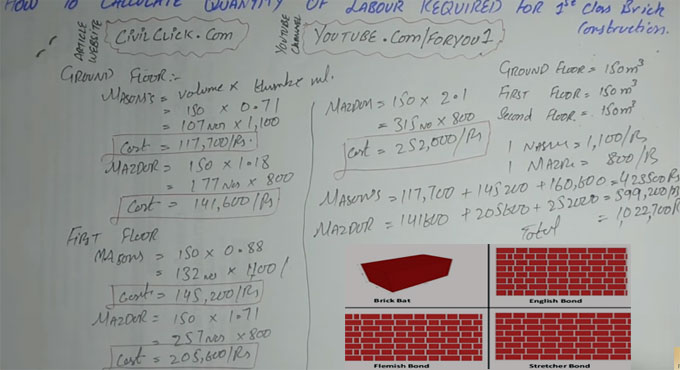# How to calculate mason, labor for brick masonry construction with help of thumb ruleIn this construction video tutorial, you will learn how to compute the mason as well as labor toward a 1st class brick masonry construction with the help of thumb rule.

A thumb rule refers to a principle having extensive application that is not projected to be exactly perfect or authentic for each situation. This method can be easily applied for making approx calculation or recollecting some value, or for making some determination.

It should be noted that the quantity of required labor will be gradually increased from ground floor to first floor to second floor etc.

Thumb rule is a constant value that is taken from the earlier construction work i.e. how much volume is covered by the labors employed in earlier projects. All the volumes are summed up to find out the average volume.

In ground floor, first floor and second floor, the volume of total area is taken as 150 m3

Ground floor :-

Mason = Volume x thumb rule (for mason) = 150 x 0.71 = 107 numbers
Now, suppose the charge for one mason = 1100 rupees
So, the cost for mason will be 107 x 1100 = 117, 700 rupees

Labor = 150 x 1.18 (thumb rule for labor) = 177 numbers
Suppose, one labor charge 800 rupees
Therefore, cost of labor = 177 x 800 = 141,600 rupees

Similar process should be followed for 1st floor and 2nd floor. Here, the value of thumb rule will be increased as the steps are increase.

To learn the calculation for 1st and 2nd floor, watch the following construction video tutorial.

Video Source: F&U-FORYOU